# KSEEB Solutions for Class 9 Science Chapter 8 Motion

In this chapter, we provide KSEEB SSLC Class 9 Science Chapter 8 Motion for English medium students, Which will very helpful for every student in their exams. Students can download the latest KSEEB SSLC Class 9 Science Chapter 8 Motion pdf, free KSEEB SSLC Class 9 Science Chapter 8 Motion pdf download. Now you will get step by step solution to each question.

### KSEEB Solutions for Class 9 Science Chapter 8 Intext Questions

KSEEB Solutions For Class 9 Science Question 1.
An object has moved through a distance can it have zero displacement? If yes, support your answer with an example.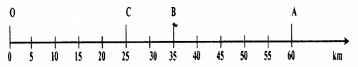In the figure distance of an object moved from 0 to A is 60 km and distance travelled is 60 km and displacement is 60 km. From 0 to A and back to B, distance travelled is 60 km + 25 km = 85 km. But displacement (35 km) is not equal to distance travelled (85 km). If we observe like this, displacement of a body is zero, but distance travelled is not zero, starting from point ‘0’ and returning back to ‘O’ final position is mixing with initial point. Hence displacement is zero.

KSEEB Solutions For Class 9 Science Motion Question 2.
A farmer moves along the boundary of a square field of side 10 m in 40s. What will be the magnitude of displacement of the farmer at the end of 2 minutes 20 seconds from his initial position?
A farmer moves along the boundary of a square field of side 10 m in 40s
2 minutes 20 seconds means 140 seconds.
Distance travelled by farmer
= 4040 × 140 m
= 140 m.
∴ Farmer moves for 2 minutes 20 seconds. It means 14040 = 3.5 rounds
From the original point, he moves for 2 minutes 20 seconds.
Case i)
Original point means any point in the corner of the field. In this case he moves for 2 min 20 seconds from the diagonal of the field.
Displacement is equal to diagonal of the field displacement
= 102+102−−−−−−−−√
= 14.1 m.
Case ii)
Original point means any point in the middle of the side of the field.
∴ Displacement is equal to any side of the field = 10 m.
It means displacement is between any original point ie in between 14.1 m and 10 m.

KSEEB Solutions For Class 9 Science Chapter 8 Question 3.
Which of the following is true for displacement?
a) It cannot be zero.
b) Its magnitude is greater than the distance travelled by the object.
b) Its magnitude is greater than the distance travelled by the object.

KSEEB Solutions For Class 9th Science Question 4.
Distinguish between speed and velocity

KSEEB Solutions For Class 9 Physics Question 5.
Under what condition (s) is the magnitude of the average velocity of an object equal to its average speed?
If the speed of the object changing uniformly, the magnitude of the average velocity of an object equal to its average speed.

KSEEB Solutions Class 9 Science Question 6.
What does the odometer of an automobile measure?
Odometer measures the speed of the vehicle.

KSEEB 9th Science Solutions Question 7.
What does the path of an object look like when it is in uniform motion?
When the object is in uniform motion, it covers equal distance at equal intervals of time.

KSEEB Solutions For Class 9 Question 8.
During an experiment, a signal from a spaceship reached the ground station in five minutes. What was the distance of the spaceship from the ground station? The signal travels at the speed of light, which is 3 × 10 ms-1.
Time taken by the signal to reach the ground station from the spaceship = 5 min = 5 × 60 = 300 seconds
Speed of the signal = 3 × 108 m/s.
Speed =  Total distance travelled  Total time taken
∴ Distance travelled = speed × time taken
= 3 × 108 × 300
= 9 × 1010m
∴ Distance of change of velocity = 9 × 1010 m

KSEEB Solutions For 9th Class Science Question 9.
When will you say a body is in

1. Uniform acceleration?
2. non-uniform acceleration?

1. If the object covers equal distances in equal intervals of time, it is added to be in uniform motion.
2. If the object covers unequal distances in equal intervals of time, it is said to be in non-uniform motion.

KSEEB Solutions For 9 Science Question 10.
A bus decreases its speed from 80 km h-1 to 60 km h-1 in 5s. Find the acceleration of the bus.
Initial velocity of the bus u = 80 kmh-1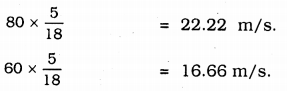Final velocity, V = 60 km/h
time taken to decrease the velocity of bus, t = 5 seconds
acceleration, a = 5 seconds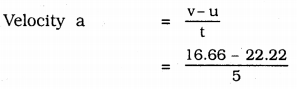= -1.112 ms-2.

KSEEB Solutions Science Class 9 Question 11.
A train starting from a railway station and moving with uniform acceleration attains a speed 40 km h-1 in 10 minutes. Find its acceleration.
Initial speed of the train u = 0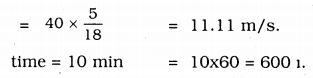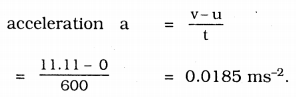∴ acceleration of a train = 0.0185 ms-2.

KSEEB Solutions 9 Science Question 12.
What is the nature of the distance-time graphs for uniform and non-uniform motion of an object?
(i) The nature of the distance-time graphs for the uniform motion of an object is a straight line.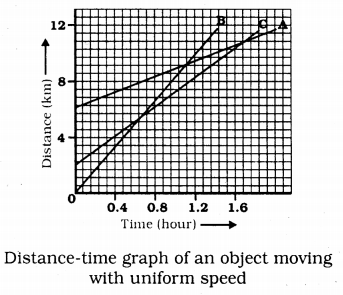(ii) The nature of tire distance time graph for non-uniform motion is curved line.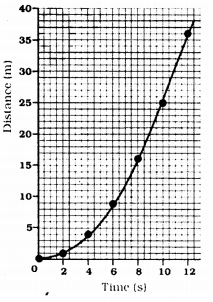Distance-time graph for a car moving with non-uniform speed

KSEEB Solutions For 9th Science Question 13.
What can you say about the motion of an object whose distance-time graph is straight line parallel to the time axis?
This graph indicates the object is at rest.

KSEEB Science Solutions For Class 9 Question 14.
What can you say about the motion of an object if its speed-time graph is a straight line parallel to the time axis?
This graph indicates the object is in uniform motion.

KSEEB Solutions For Science Class 9 Question 15.
What is the quantity which is measured by the area occupied below the velocity-time graph?
The quantity which is measured by the area occupied below the velocity-time graph is length = l

Science KSEEB Solutions 9 Question 16.
A bus starting from rest moves with a uniform acceleration of 0.1 ms-2 for 2 minutes. Find
(a) the speed acquired,
(b) the distance travelled.
(a) Initial velocity of the bus u = 0 (Bus is at rest).
acceleration, a = 0.1 ms-2
time t = 2 minutes = 120 seconds
Let the speed of bus be ‘V’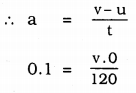∴ v = 12m/s
(b) As per third law of motion:
v2 – u2 = 2as
(12)2 – (0)2 = 2(0. 1)s
∴ s = 720 m.
the speed of acquired by bus = 12 m/s.
the distance travelled = 720 m.

9th Standard Science Textbook State Syllabus Question 17.
A train is travelling at a speed of 90 kmh-1 Brakes are applied so as to produce a uniform acceleration of -0.5 ms-2. Find how far the train will go before it is brought to rest.
Let the initial speed of the train be u = 90 Km/h
= 25 m/s.
Final speed of the train, v = 0 (train comes to rest)
acceleration a = 0.5 ms-2
As per 3rd law of motion
v2 = u2 + 2as
(0)2 = (25)2 + 2(0.5)s
s = train travelled distance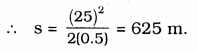Train will travel 625 km before it is brought to rest.

KSEEB Solution For Class 9 Science Question 18.
A trolley, while going down an inclined plane has an acceleration of 2cms-2 what will be its velocity 3s after the start?
Initial velocity of a trolley, u = 0 (at rest)
acceleration, a = 2 cms-2 = 0.02 m/s2 time t = 3S
As per 1 st law of motion
v = u + at
Here V means velocity of a trolley after 3 s
V = 0 + 0.02 × 3
= 0.06 m/s.
∴ = 0.06 m/s.

KSEEB Solutions For Class 9 Science Force And Laws Of Motion Question 19.
A racing car has a uniform acceleration of 4ms-2, what distance will it cover in 10s after start?
Initial velocity of a racing car, u = 0 (at rest)
acceleration, a = 4 m/s2
time t = 10 s
As per second law of motion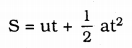Here S means distance travelled by car after 10S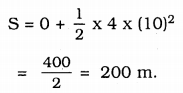∴ Distance covered by car after 10 S = 200 m.

KSEEB Class 9 Science Question 20.
A stone is thrown in a vertically upward direction with a velocity of 5ms-1 If the acceleration of the stone during its motion is 10ms-2 in the downward direction, what will he the height attain by the stone and how much time will it take to reach there?
The initial velocity of a stone, u = 5 ms-1.
Final velocity of a stone v = 0 (at rest)
If the acceleration of the stone during its motion is = -10ms-2.
Let the maximum height be ‘s’
v = u + at
0 = 5 + (-10)t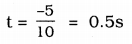time taken by stone = 0.5s
As per 3rd Law of motion
v2 = u2 + 2as
(0)2 = (5)2 + 2(-10)s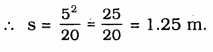The height attained by the store = 1.25 m.

### KSEEB Solutions for Class 9 Science Chapter 8 Textbook Exercises

9th Standard Science Notes State Syllabus Question 1.
An athlete completes one round of a circular track of diameter 200 m. in the 40s. What will be the distance covered and the displace¬ment at the end of 2 minutes 20s?
Diameter of circular track, d = 200 m.
r = d2 = 100m
Circumference = 2πr = 2p(100) = 200πcm.
in 40s, athlete covers distance 200πm
in 1s, distance covered by athlete = 200π40 m
Athlete runs for 2 minutes 20s means 140s
∴ Displacement of all athlete is equal to the diameter of circle
= 200×2240×7 × 140
= 2200 m
∴ Displacement = 200 m.

Joseph jogs from one end A to the other end B of a straight 300 M road in 2 minutes 30 seconds and then turns around and jogs 100 M back to point C in another 1 minute. What are Joseph’s average speeds and velocities in jogging (a) from A to B and (b) from A to C?
a) from A to B distance covered by Joseph = 300 M.
Time taken to cover this distance
= 2 min 30 sec. = 150 seconds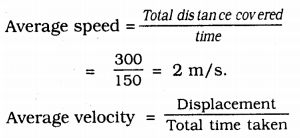Displacement = Nearest distance between A and B = 300 m.
Time taken = 150s
Average velocity = ygy = 2 m/s.

b) From A to C :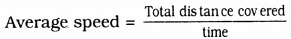Total distance covered = Distance between A to B + distance between B to C
= 300 + 100 = 400 m.
Total time taken to cover from A to B + Time taken to cover from B to C
= 150 + 60= 210s.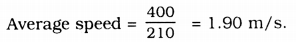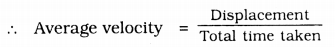Displacement from A to C
= AC + AB – BC
= 300 – 100
= 200m
Total time taken = Time taken to travel from A to B + Time taken to travel from B to C
= 210 s
∴ Average velocity = 200210 =0.952 ms-1 .

9th Standard Kannada Medium Science Guide Question 3.
Abdul, while during to school, computes the average speed for his trip to be 20Kmh-1 on his return trip along the some route, there is less traffic and the average speed is 30 Kmh-1. What is the average speed for Abdul’s trip?
i) Average speed of Abdul’s trip while driving to school = 20 Kmh-1
Average speed =  Total distance  Total time taken
Total distance = Distance travelled to reach school = t1
∴ 20 = dt1
t1 = d20 …………… (i)
While returning from school
Total distance = Distance travelled while returning from school = d Now total time taken = t2.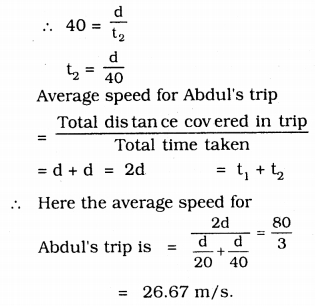KSEEB Solutions 9th Science Question 4.
A motorboat starting from rest on a lake accelerates in a straight line at a constant rate of 3.0 ms-2 for 8.0 s. How far does the boat travel during this time?
Initial velocity of motor boat, u =0
(at rest) ,
Accieratlon of a motor boat a = 3m/s2
Time taken, t = 85
According to second equation of motion, s
S = 0 +12 × (8)= 96m.
∴ Motorboat travels = 96m.

9th Science Notes KSEEB Question 5.
A driver of a car travelling at 52kmh-1 applies the brakes and accelerates uniformly in the opposite direction. The car stops in 5s. Another driver going at 3kmh-1 in another car applies his brakes slowly and stop In 10s. on the same graph paper. Plot the speed versus time graphs for the two cars, which of the two cars travelled farther after the brakes were applied?
initial velocity of A car u1 = 52 kmh-1
= 14.4 m/s.
Time taken to stop car t1 = 5 s.
After 5s car comes to rest Initial velocity of B car, u2 = 3Kmh-1
= 0.833 m/s. = 0.83 m/s.
Time taken to stop car t2 = 10s
After 10s car comes to rest.
Graph: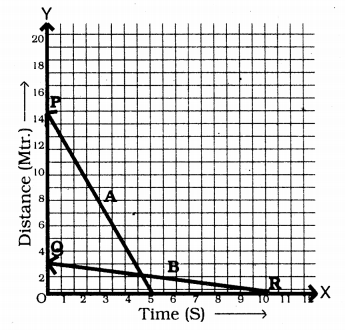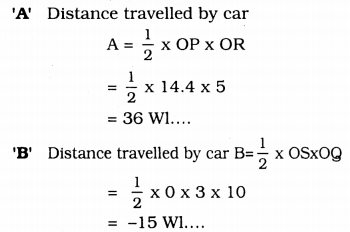Area of ∆OPR = Area of ∆OSQ
When compared car A to car B, A car travelled father. After brake it travels 52 Kmh-1

KSEEB 9th Class Science Solutions Question 6.
Fig 8.11 shows the distance-time graph of three objects A, B and C. Study the graph and answer the following questions.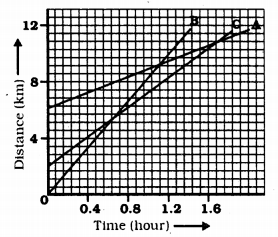a) Which of the three is travelled the fastest?
b) Are all three ever at the same point on the road?
c) How far has C travelled when B passes A?
d) How far has B travelled by the time passes C?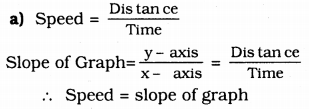Slope of B object is greater than slopes A and C. This is travelling fastest.

b) All three objects never meet at a point, hence these are not at the same point.

c) There are 7 boxes in X-axis = 4 Km
1 box = 47 Km
In the beginning, C object is 4 boxes away from 0
= 167 km
Distance to C from origin = Distance of C when it moves from B to A =8 Km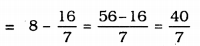∴ Distance travelled by C when B passes A = 5.714 km.

d) Distance B travelled by the time it passes C = 9 boxes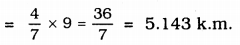∴ B has travelled 5.143 k.m. by the time when it passes C.

9th Standard Science Question Answer Question 7.
A ball is gently dropped from a height of 20 m. If its velocity increases uniformly at the rate of 10ms-1 with what velocity will it strike the ground? After what time will it strike the ground?
Distance ball covers, S = 20 m
acceleration, a = 10Kmh-1 = 10 m/s
initial velocity, u = 0 (at rest)
When ball strikes, its final velocity,
According to 3rd law of motion
v2 = u2 + 2as
v2 = 0 + 2(10)(20)
v = 20 m/s.
According to 1 st Law of motion v = u + at
Time taken by ball to strike the ground, t,
20 = 0 + 10 (t)
∴ t = 2s
∴ 2s is required to ball for striking the ground and velocity is 20 m/s

9th Science KSEEB Solutions Question 8.
The speed-time graph for a car is shown in figure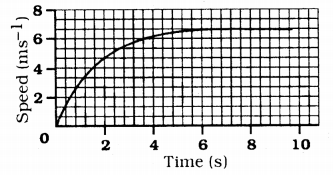a) Find how far does the car travel in the first 4 seconds. Shade the area on the graph that represents the distance travelled by car during the period.
b) Which part of the graph represents uniform motion of the car?
a) Shade lightly in the graph about distance travelled by car in 4s.
b) Shade with red colour about 6 cm to 10 cm. It represents the uniform motion of the car.

Karnataka Solutions For Class 9 Science Question 9.
State which of the following situations are possible and give an example for each of these;
a) an object with a constant acceleration but with zero velocity.
b) an object moving in a certain direction with an acceleration in the perpendicular direction.
a) This is possible
Eg: when a ball is thrown to a height its velocity is zero.
(For a ball G = 9.8 m/s2)

b) This is possible
Eg: when a car moves in a curved line, its acceleration is perpendicular to the given direction)

9th State Syllabus Science Notes Question 10.
An artificial satellite is moving in a circular orbit of radius 42250 km. Calculate its speed if it takes 24 hours to revolve around the earth.
Satellite is moving in circular orbit radium of satellite r = 42250 km
time t = 24 Hrs = 3600 × 24 S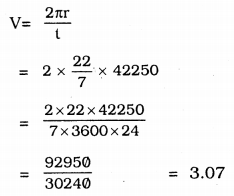∴ Speed of the satellite when it moves in circular orbit = 3.07 kms-1.

### KSEEB Solutions for Class 9 Science Chapter 8 Additional Questions

Fill in the blanks with suitable words.

KSEEB Solutions 9th Class Science Question 1.
The two physical quantities required to know final position of an object are _________ and _________
Distance travelled, displacement.

9th Standard Science Notes Pdf In Kannada Question 2.
S.I. unit of speed is ________
m/s

9th Class Science Notes In Kannada Pdf Question 3.
Distance travelled by the object in unit time is ________
Speed.

KSEEB 9th Science Question 4.
If acceleration is in the direction of velocity it is _________ acceleration.
positive.

9th Science Solutions KSEEB Question 5.
When an object moves in a circular path with uniform speed, its motion is called ________
uniform circular motion.

KSEEB Solutions For Class 9 Science Physics Question 1.
Give an express10n for centripetal force?
f=mv2r

9th Standard Science Notes Question 2.
What is uniform circular mot10n?
Body moving in a circular path arbitrary any instant along a tangential to the posit10n of the body on the circular path at that instant or time.

A body falls freely. What is constant?
When the body falls freely. It has a constant acceleration.

Define uniform speed.
The speed of an object is said to be uniform speed if it travels equal distances in equal intervals of time.

All Chapter KSEEB Solutions For Class 9 Science

—————————————————————————–

All Subject KSEEB Solutions For Class 9

*************************************************

I think you got complete solutions for this chapter. If You have any queries regarding this chapter, please comment on the below section our subject teacher will answer you. We tried our best to give complete solutions so you got good marks in your exam.

If these solutions have helped you, you can also share kseebsolutionsfor.com to your friends.

Best of Luck!!